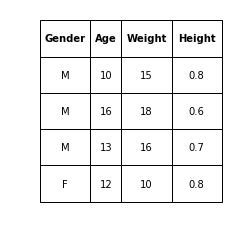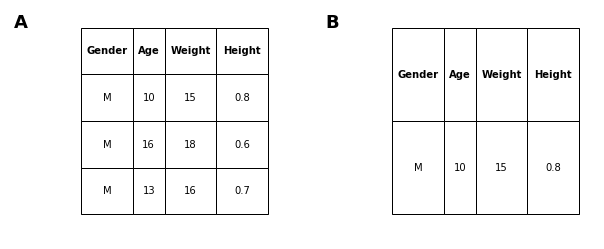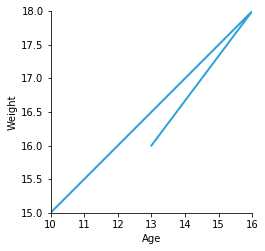# Table¶

Title
Table Element
Dependencies
Matplotlib
Backends
Matplotlib
Bokeh
In :
import holoviews as hv
hv.extension('matplotlib')A table is more general than an ItemTable, as it allows multi-dimensional keys and multidimensional values. Let's say we have the following data:

In :
gender = ['M','M', 'M','F']
age = [10,16,13,12]
weight = [15,18,16,10]
height = [0.8,0.6,0.7,0.8]


We can construct a Table using a dictionary format (identical in format as that accepted by the pandas DataFrame):

In :
hv.Table({'Gender':gender, 'Age':age, 'Weight':weight, 'Height':height},
['Gender', 'Age'], ['Weight', 'Height'])

Out:Or we can declare the same table by dimension position, with key dimensions followed by value dimensions:

In :
table = hv.Table((gender, age, weight, height), ['Gender', 'Age'], ['Weight', 'Height'])
table

Out:Note that you can use the select method using tables by the key dimensions:

In :
table.select(Gender='M') + table.select(Gender='M', Age=10)

Out:The Table is used as a common data structure that may be converted to any other HoloViews data structure via the to utility available on the object. Here we use this utility to show the weight of the males in our datset by age:

In :
table.select(Gender='M').to.curve(kdims=["Age"], vdims=["Weight"])

Out:For more extended usage of table conversion see the Tabular Data user guide.

For full documentation and the available style and plot options, use hv.help(hv.Table).